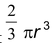# Surface area and volume class 9 all formulas

## Right circular cone

1. Curved surface area of a cone : π rh
2. Total surface area of a cone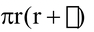3. Volume of Right Circular Cone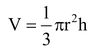### Right circular cylinder

(i) Curved surface area of a cylinder 2πrh

(ii) Total surface area of a cylinder

2π r(h+r)

(iii) Volume of cylinder : π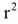h

### Cuboid and cube

(i) Total surface area of cuboid(ii) Lateral surface area of cuboid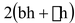(iii) Volume of cuboid : lbh

(iv) Lateral surface area of cube : 4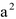(v) Total surface area of cube : 6(vi) Volume of cube :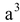### Sphere and Hemi-sphere

(i) Surface area of sphere : 4π(ii) Curved surface area of hemi-sphere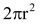(iii) Total surface area of hemi-sphere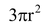(iv) Volume of sphere :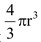(v) Volume of hemi-sphere